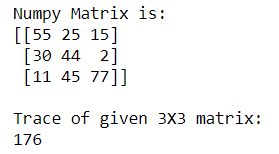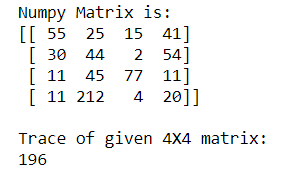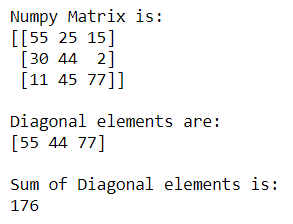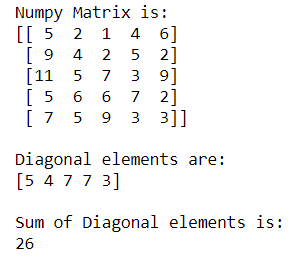# Calculate the sum of the diagonal elements of a NumPy array

• Last Updated : 05 Sep, 2020

Sometimes we need to find the sum of the Upper right, Upper left, Lower right, or lower left diagonal elements. Numpy provides us the facility to compute the sum of different diagonals elements using numpy.trace() and numpy.diagonal() method.

Method 1: Finding the sum of diagonal elements using numpy.trace()

Attention geek! Strengthen your foundations with the Python Programming Foundation Course and learn the basics.

To begin with, your interview preparations Enhance your Data Structures concepts with the Python DS Course. And to begin with your Machine Learning Journey, join the Machine Learning - Basic Level Course

Syntax : numpy.trace(a, offset=0, axis1=0, axis2=1, dtype=None, out=None)

Example 1: For 3X3 Numpy matrix

## Python3

 `# importing Numpy package``import` `numpy as np`` ` `# creating a 3X3 Numpy matrix``n_array ``=` `np.array([[``55``, ``25``, ``15``],``                    ``[``30``, ``44``, ``2``],``                    ``[``11``, ``45``, ``77``]])`` ` `# Displaying the Matrix``print``(``"Numpy Matrix is:"``)``print``(n_array)`` ` `# calculating the Trace of a matrix``trace ``=` `np.trace(n_array)`` ` ` ` `print``(``"\nTrace of given 3X3 matrix:"``)``print``(trace)`

Output:Example 2: For 4X4 Numpy matrix

## Python3

 `# importing Numpy package``import` `numpy as np`` ` `# creating a 4X4 Numpy matrix``n_array ``=` `np.array([[``55``, ``25``, ``15``, ``41``],``                    ``[``30``, ``44``, ``2``, ``54``],``                    ``[``11``, ``45``, ``77``, ``11``],``                    ``[``11``, ``212``, ``4``, ``20``]])`` ` `# Displaying the Matrix``print``(``"Numpy Matrix is:"``)``print``(n_array)`` ` `# calculating the Trace of a matrix``trace ``=` `np.trace(n_array)`` ` ` ` `print``(``"\nTrace of given 4X4 matrix:"``)``print``(trace)`

Output:Method 2: Finding the sum of diagonal elements using numpy.diagonal()

Syntax :

```numpy.diagonal(a, offset=0, axis1=0, axis2=1
```

Example 1: For 3X3 Numpy Matrix

## Python3

 `# importing Numpy package``import` `numpy as np`` ` `# creating a 3X3 Numpy matrix``n_array ``=` `np.array([[``55``, ``25``, ``15``],``                    ``[``30``, ``44``, ``2``],``                    ``[``11``, ``45``, ``77``]])`` ` `# Displaying the Matrix``print``(``"Numpy Matrix is:"``)``print``(n_array)`` ` `# Finding the diagonal elements of a matrix``diag ``=` `np.diagonal(n_array)`` ` `print``(``"\nDiagonal elements are:"``)``print``(diag)`` ` `print``(``"\nSum of Diagonal elements is:"``)``print``(``sum``(diag))`

Output:Example 2: For 5X5 Numpy Matrix

## Python3

 `# importing Numpy package``import` `numpy as np`` ` `# creating a 5X5 Numpy matrix``n_array ``=` `np.array([[``5``, ``2``, ``1``, ``4``, ``6``],``                    ``[``9``, ``4``, ``2``, ``5``, ``2``],``                    ``[``11``, ``5``, ``7``, ``3``, ``9``],``                    ``[``5``, ``6``, ``6``, ``7``, ``2``],``                    ``[``7``, ``5``, ``9``, ``3``, ``3``]])`` ` `# Displaying the Matrix``print``(``"Numpy Matrix is:"``)``print``(n_array)`` ` `# Finding the diagonal elements of a matrix``diag ``=` `np.diagonal(n_array)`` ` `print``(``"\nDiagonal elements are:"``)``print``(diag)`` ` `print``(``"\nSum of Diagonal elements is:"``)``print``(``sum``(diag))`

Output:My Personal Notes arrow_drop_up